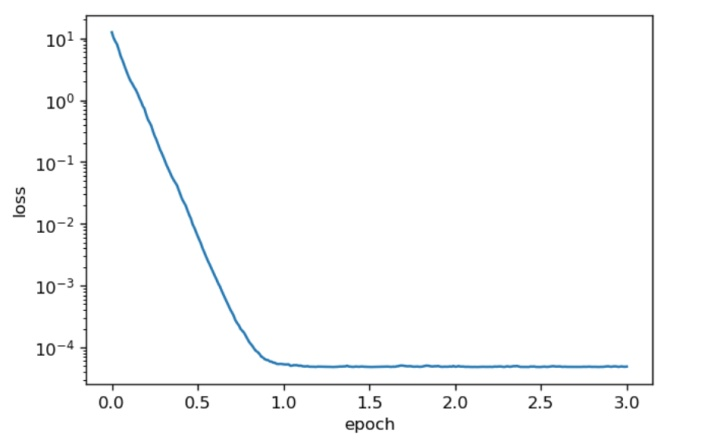GiantPandaCV导语：这篇文章的内容主要是参考 沐神的mxnet/gluon视频中，Aston Zhang讲解的优化部分 对深度学习调参背后的数学原理进行讲解。文章有些长，小伙伴们可以耐心读完，相信收获一定会很大。

# 1. 前言¶

1. 设计模型
2. 构造loss function
3. 通过优化算法，找到一个某种意义上的optim值

# 2. 调参背后的数学原理¶

1. 定义一个损失函数 loss function
2. 通过优化算法来最小化这个loss function
3. 找到使得loss function最大或者最小的optim解作为model的parameter值

# 3. 从梯度下降说起¶

• 首先假设我们要学习的参数是$x$
• 那么，$x := x-\eta \frac{\partial Loss}{\partial x}$
• 这里的$x$$loss$都是一维实数集上的一个映射$f(x-\eta f'(x)) \approx f(x)-\eta f'(x)f’(x)+O(\epsilon^2)$

$f(x-\eta f'(x)) \approx f(x)-\eta f'(x) f’(x)$

• 注意到$[f'(x)]^2$是一个大于等于0的数
• $\eta$也是一个大于0的数，那么$\eta f'(x) f’(x)$ 也是一个大于等于0的数
• $x-\eta f'(x)$ 可以类比到开始我们讲过的公式 $x := x-\eta \frac{\partial Loss}{\partial x}$

• 那么，此时式子又可以被简化为：$f(x-\eta f'(x)) \leq f(x)$

• $f'(x)$固定的时候，如果$\eta$是一个非常大的数值的时候，那么此时的$\epsilon$ 就不能满足非常小的定义。那么，这个时候$O(\epsilon^2)$ 这一项就不能被drop掉，如果不能被drop掉，就不能满足泰勒展开的基本定义。这也就对应了我们在训练的过程中，如果把learning_rate（此处的$\eta$对应到learning_rate）调的太大，就出现了NaN的情况。
• $\eta$是一个固定的很小值的时候，如果$x$的初始值选择了一个很大的数值，比如说，这里$f(x)=x^2$，那么此时的$f'(x) = 2x$, $[f'(x)]^2=4x^2$，这一项的数值也会变得非常大。导致其不能满足$\epsilon$ 不是一个非常小的数值的条件。此时训练肯定也会出问题。

1. 学习率的大小选择
2. 初始化参数值的选择

# 4. 学习率¶

## 4.1 当我们 学习率太小的时候：¶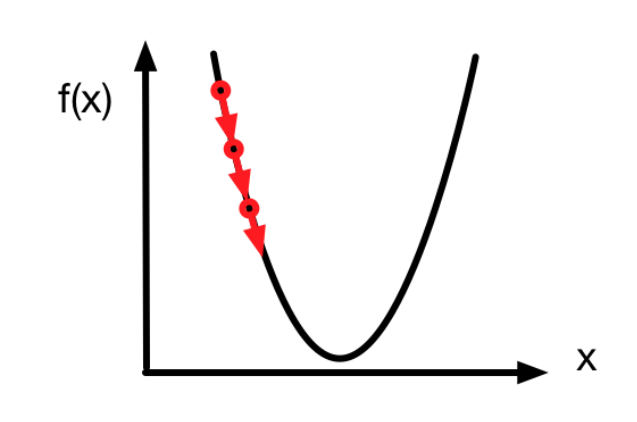## 4.2 当我们的学习率设置的过大¶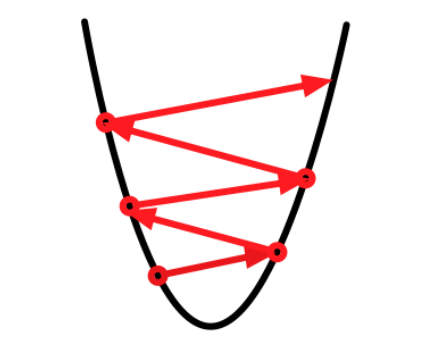# 5. 随机梯度下降¶

• 先来看看我们优化问题的目标函数$f(x) = \frac{1}{n}\sum_{i=1}^nf_i(x)$
• 其中$f_i(x)$表示的是第$i$个样本所带来的损失
• 可以观察到，梯度下降每次进行迭代的开销随着n的增长呈线性增长。
• 因此，如果我们的训练样本个数非常多，那么一次迭代的开销也将非常大

$E[\frac{\\partial f_i(x)}{\partial x}] = \frac{1}{n}\sum_{i=1}^n\frac{\partial f_i(x)}{\partial x} = \frac{\partial f(x)}{\partial x}$

# 6. Mini-Batch的随机梯度下降¶

## 6.1 算法实现¶

• 当batch_size == 1的时候，就是sgd

• 当batch_size == 整个训练集大小的时候，就是梯度下降

  def sgd( params, lr, batch_size ):
for param in params:


from mxnet import gluon
from mxnet.gluon import nn
from mxnet import ndarray as nd
import mxnet as mx
from time import time
from mxnet import sym
import matplotlib.pyplot as plt
import numpy as np
import random

mx.random.seed(1)
random.seed(1)

def sgd( params, lr, batch_size ):
for param in params:

# 生成数据集
num_inputs = 2
num_examples = 1000
true_w = [2,-3.4]
true_b = 4.2
X = nd.random_normal(scale = 1,shape = (num_examples,num_inputs))
y = true_w*X[:,0] + true_w*X[:,1] + true_b
y += 0.01*nd.random_normal(scale = 1, shape = y.shape)
dataset = gluon.data.ArrayDataset(X,y)

def data_iter(batch_size):
idx = list(range(num_examples))
random.shuffle(idx)
for batch_i, i in enumerate(range(0,num_examples,batch_size)):
j = nd.array(idx[i:min(i+batch_size,num_examples)])
yield batch_i,X.take(j),y.take(j)

def init_params():
w = nd.random_normal(scale = 1,shape = (num_inputs,1))
b = nd.zeros(shape = (1,))
params = [w,b]
for param in params:
return params

def net(X,w,b):
return nd.dot(X,w)+b

def square_loss(yhat,y):
return (yhat-y.reshape(yhat.shape))**2/2

def train(batch_size, lr, epochs, period):
assert period >= batch_size and period % batch_size == 0
w, b = init_params()
total_loss = [np.mean(square_loss(net(X, w, b), y).asnumpy())]
# 注意epoch从1开始计数。
for epoch in range(1, epochs + 1):
# 学习率自我衰减。
if epoch > 2:
lr *= 0.1
for batch_i, data, label in data_iter(batch_size):
output = net(data, w, b)
loss = square_loss(output, label)
loss.backward()
sgd([w, b], lr, batch_size)
if batch_i * batch_size % period == 0:
total_loss.append(
np.mean(square_loss(net(X, w, b), y).asnumpy()))
print("Batch size %d, Learning rate %f, Epoch %d, loss %.4e" %
(batch_size, lr, epoch, total_loss[-1]))
print('w:', np.reshape(w.asnumpy(), (1, -1)),
'b:', b.asnumpy(), '\n')
x_axis = np.linspace(0, epochs, len(total_loss), endpoint=True)
plt.semilogy(x_axis, total_loss)
plt.xlabel('epoch')
plt.ylabel('loss')
plt.show()


period参数：每次采用到与period相同数目的数据点后，记录当前目标函数值用于作图。

## 6.2 调参¶

• 当batch_size = 1时，该训练方式就是sgd，当前lr的情况下，loss function在前期快速减少，当epoch > 2的时候，lr会出现自我衰减，loss function下降后基本收敛，最终学习到的parameter和真实parameter相当。
  train(batch_size = 1,lr = 0.1, epochs = 5, period = 10)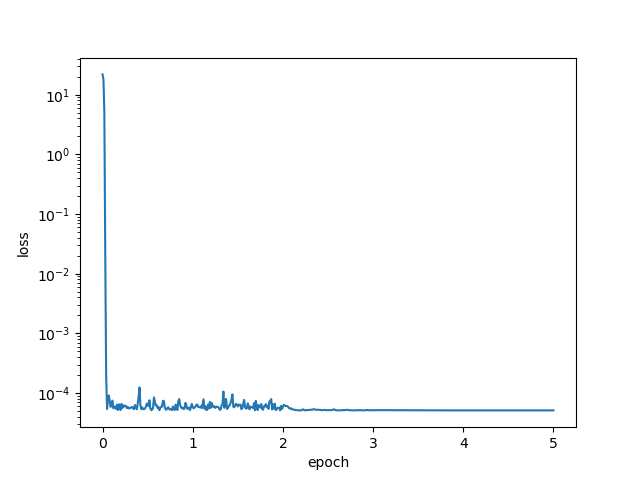• 当batch_size = 1000时，由于训练数据集包含1000个样本，此时训练使用的是标注的梯度下降算法。loss function还是在前两个epoch的时候下降较快。当epoch > 2的时候，lr自我衰减。loss function下降缓慢。最终学习到的parameter和真实parameter相当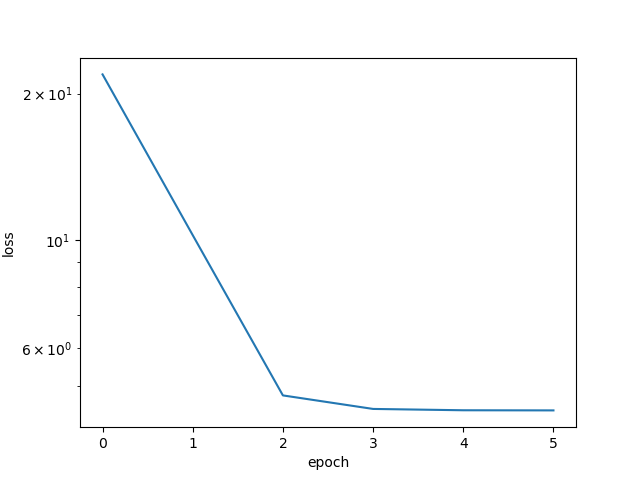• 当batch_size = 10时，由于训练样本中含有1000个样本，此时训练使用mini-batch的sgd来进行。最终学到的parameter与真实parameter相当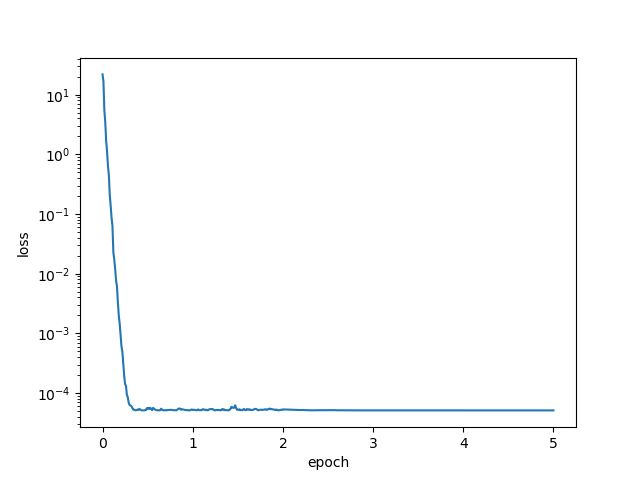train(batch_size = 10, lr = 3, epochs = 5, period = 10)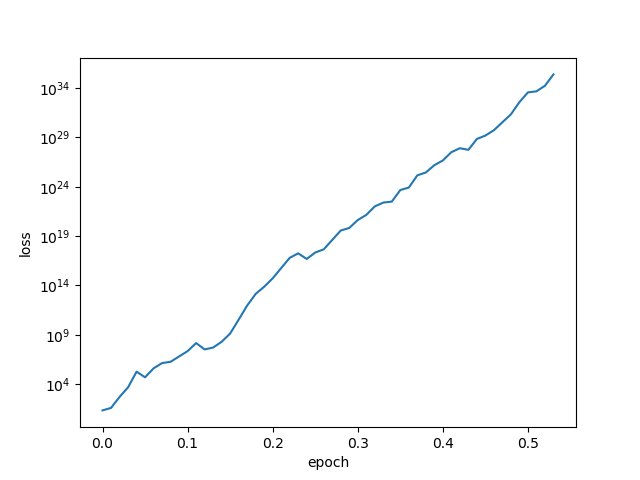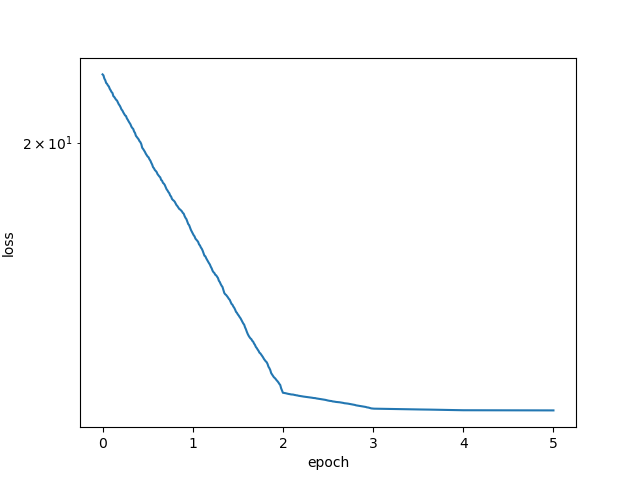## 7. 动量法 momentum¶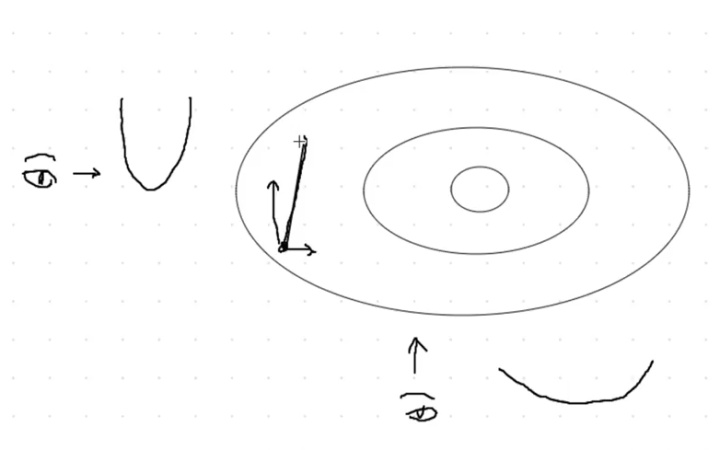• 如果我们是从最左边的眼睛看，那么会看到一个变化非常“陡峭”的部分
• 如果我们是从最下面的眼睛看，那么会看到一个相对“平缓”的部分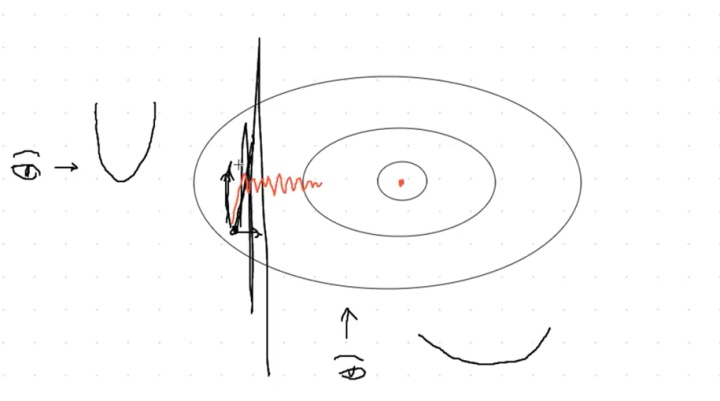• 如果lr选择的过大，就会出现overshot问题，模型loss出现nan，黑色线条部分
• 如果lr选择的过小，就会出现undershot问题，迭代多步仍不收敛，红色线条部分

$v := \gamma v + \eta \frac{\partial f}{\partial x}$

$x := x - v$

$v := \gamma v + (1-\gamma) \frac{\eta}{1-\gamma} \frac{\partial f}{\partial x}$

$y^{(t)} = \gamma y^{(t+1)}+(1-\gamma)x^{(t)}$$\gamma = 0.95，t=20$• 随着迭代的进行，$x$的指数越大，那么前面的系数就越小
• $x$的指数越接近20的项，其前面的系数就越大

$v := \gamma v+\eta \frac{\partial f}{\partial x}$ $v := \gamma v + (1-\gamma) \frac{\eta}{1-\gamma} \frac{\partial f}{\partial x}$

$y^{(t)} = \gamma y^{(t+1)}+(1-\gamma)x^{(t)}$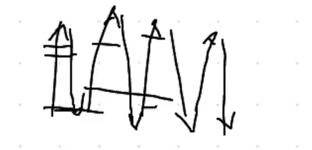• 出现overshot的问题的时候，momentum方法可以对其进行一个裁剪。使得其在方差过大的走偏情况下，能够尽量削弱这种情况，得到尽可能小的方差
• 出现undershot的问题的时候，momentum方法可以对其进行一个加速，使得这种趋势能够加强。

import mxnet as mx
from mxnet import gluon
from mxnet import ndarray as nd
import numpy as np
import random

mx.random.seed(1)
random.seed(1)

# 生成数据集。
num_inputs = 2
num_examples = 1000
true_w = [2, -3.4]
true_b = 4.2
X = nd.random_normal(scale=1, shape=(num_examples, num_inputs))
y = true_w * X[:, 0] + true_w * X[:, 1] + true_b
y += .01 * nd.random_normal(scale=1, shape=y.shape)
dataset = gluon.data.ArrayDataset(X, y)

net = gluon.nn.Sequential()
square_loss = gluon.loss.L2Loss()
%matplotlib inline
import matplotlib as mpl
mpl.rcParams['figure.dpi']= 120
import matplotlib.pyplot as plt

def train(batch_size, lr, mom, epochs, period):
assert period >= batch_size and period % batch_size == 0
net.collect_params().initialize(mx.init.Normal(sigma=1), force_reinit=True)
# 动量法。
trainer = gluon.Trainer(net.collect_params(), 'sgd',
{'learning_rate': lr, 'momentum': mom})
total_loss = [np.mean(square_loss(net(X), y).asnumpy())]

for epoch in range(1, epochs + 1):
# 重设学习率。
if epoch > 2:
trainer.set_learning_rate(trainer.learning_rate * 0.1)
for batch_i, (data, label) in enumerate(data_iter):
output = net(data)
loss = square_loss(output, label)
loss.backward()
trainer.step(batch_size)

if batch_i * batch_size % period == 0:
total_loss.append(np.mean(square_loss(net(X), y).asnumpy()))
print("Batch size %d, Learning rate %f, Epoch %d, loss %.4e" %
(batch_size, trainer.learning_rate, epoch, total_loss[-1]))

print('w:', np.reshape(net.weight.data().asnumpy(), (1, -1)),
'b:', net.bias.data().asnumpy(), '\n')
x_axis = np.linspace(0, epochs, len(total_loss), endpoint=True)
plt.semilogy(x_axis, total_loss)
plt.xlabel('epoch')
plt.ylabel('loss')
plt.show()


train(batch_size=10, lr=0.2, mom=0.9, epochs=5, period=10)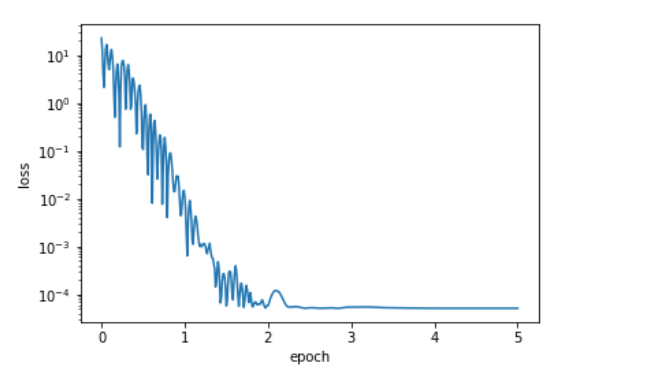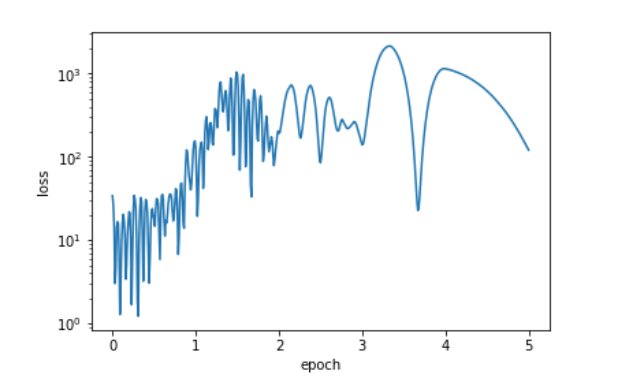$x_1 := x_1 -\eta \frac{\partial f}{\partial x_1}$

$x_2 := x_2 -\eta \frac{\partial f}{\partial x_2}$

• 使用一个梯度按元素平方的累加变量$s:=s+g \odot g$
• 其中$g$就是通过mini-batch的计算得到的梯度
• 然后通过下面的式子对模型中每个参数的学习率通过按照元素重新调整$g':=\frac{\eta}{\sqrt{s+\epsilon}}\odot g$
• 其中$\eta$ 是初始学习率，$\epsilon$是为了维持数值稳定性而添加的元素，防止分母除以0
• 然后再通过$x:=x-g'$对相应的parameter进行了更新

Adagrad算法的核心思想：我们注意到$s$其实是一个累加项的过程，

• 如果loss function相对于某一个parameter的偏导数一直都很大，那么就让他下降的快一点。
• 如果loss function相对于某一个parameter的偏导数一直都比较小，那么就让他下降的慢一点。

def adagrad( params, sqrs, lr, batch_size):
eps_stable = 1e-7
for param, sqr in zip(params,sqrs):
sqr[:] += nd.square(g)
div = lr*g/nd.sqrt(sqr+eps_stable)
param[:] -= div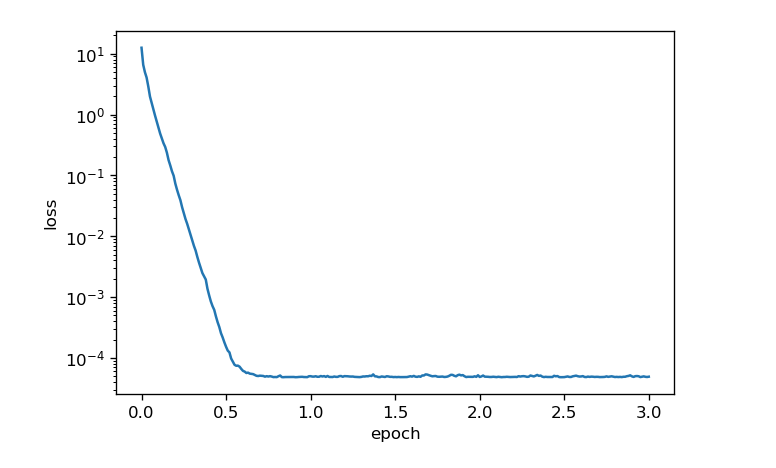# 9. RMSProp¶

def rmsprop(params, sqrs, lr, gamma, batch_size):
eps_stable = 1e-8
for param, sqr in zip(params, sqrs):
sqr[:] = gamma * sqr + (1. - gamma) * nd.square(g)
div = lr * g / nd.sqrt(sqr + eps_stable)
param[:] -= div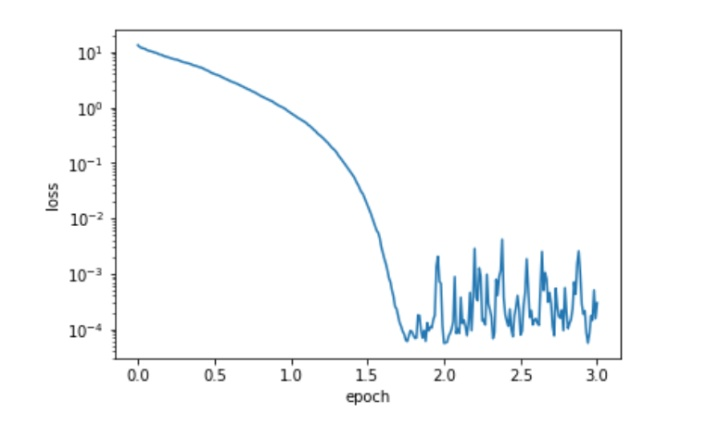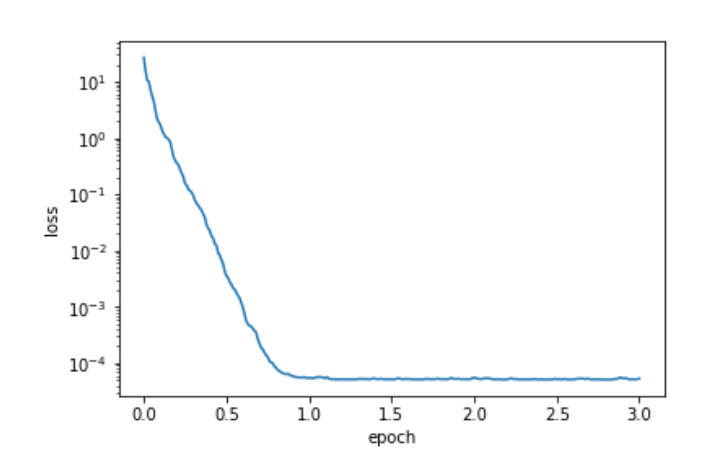• $t = t + 1$每次的迭代
• $v:=\beta_1v+(1-\beta_1)v$
• $s:=\beta_2s+(1-\beta_2)g \odot g$

• $\hat{v}:=\frac{v}{1-\beta^t_1}$
• $\hat{s}:=\frac{s}{1-\beta^t_2}$

• $g':=\frac{\eta \hat{v}}{\sqrt{\hat{s}+\epsilon}}$
• $x:=x-g'$

def adam(params, vs, sqrs, lr, batch_size, t):
beta1 = 0.9
beta2 = 0.999
eps_stable = 1e-8
for param, v, sqr in zip(params, vs, sqrs):
v[:] = beta1 * v + (1. - beta1) * g
sqr[:] = beta2 * sqr + (1. - beta2) * nd.square(g)
v_bias_corr = v / (1. - beta1 ** t)
sqr_bias_corr = sqr / (1. - beta2 ** t)
div = lr * v_bias_corr / (nd.sqrt(sqr_bias_corr) + eps_stable)
param[:] = param - div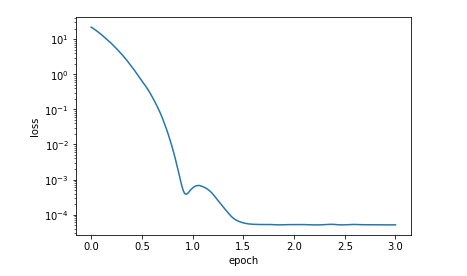• 首先$s:=\rho s+(1-\rho)g \odot g$
• 然后计算需要更新的parameter的变化量：$g' = \frac{\sqrt{\Delta x+\epsilon}}{\sqrt{s+\epsilon}}\odot g$
• 这里相比前面的算法出现了$\Delta x$ ，初始化为零张量。
• 且其计算表达式为$\Delta x := \rho \Delta x + (1-\rho)g' \odot g'$
• $x:=x-g'$
• $\rho$的取值一般在0.9~0.999范围内，当然也可以根据情况来调

def adadelta(params, sqrs, deltas, rho, batch_size):
eps_stable = 1e-5
for param, sqr, delta in zip(params, sqrs, deltas):
sqr[:] = rho * sqr + (1. - rho) * nd.square(g)
cur_delta = nd.sqrt(delta + eps_stable) / nd.sqrt(sqr + eps_stable) * g
delta[:] = rho * delta + (1. - rho) * cur_delta * cur_delta
param[:] -= cur_delta


$\rho = 0.999$，batch_size = 10时候的loss曲线图